# Using Matplotlib For Simple Plots (10/25/2019)¶

In :
import matplotlib.pyplot as plt
import pandas as pd
import numpy as np


## 1. Data Visualization with Pandas¶

The pandas package allows you to plot certain graphs with your data frames. This is one of the simplest techniques of visualizaing data. One huge benefit of using pandas is that it's super quick and requires less input from the user! First, let's plot some univariate data.

In :
iris = pd.read_csv("Iris.csv")

Out:
Id SepalLengthCm SepalWidthCm PetalLengthCm PetalWidthCm Species
0 1 5.1 3.5 1.4 0.2 Iris-setosa
1 2 4.9 3.0 1.4 0.2 Iris-setosa
2 3 4.7 3.2 1.3 0.2 Iris-setosa
3 4 4.6 3.1 1.5 0.2 Iris-setosa
4 5 5.0 3.6 1.4 0.2 Iris-setosa
In :
#Let's get a histogram of the Speal Length
iris.SepalLengthCm.hist()
plt.show()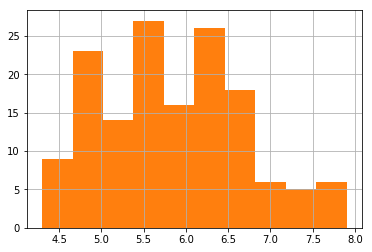In :
#Let's get the histogram of Petal Length cm
iris.PetalLengthCm.hist()
plt.show()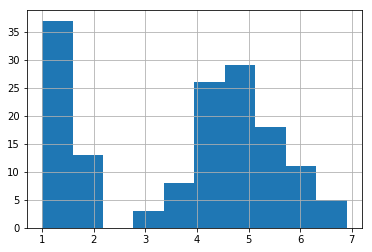In :
#Repeat with Petal Width Cm:
iris.PetalWidthCm.hist()
plt.show()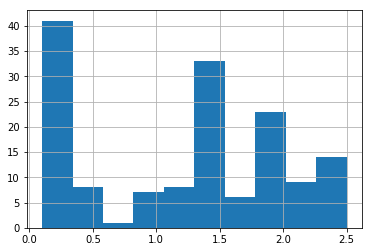In :
#Create boxplot:
iris.PetalWidthCm.plot.box()
plt.show()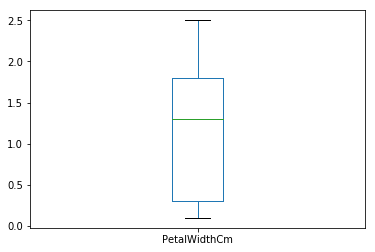In :
#Get histogram of Iris-setosa by Petal Width
#When filtering, decide what unique value you want to examine, and what particular continuous variable you choose to display.
iris[iris.Species == 'Iris-setosa']['PetalWidthCm'].hist()
plt.show()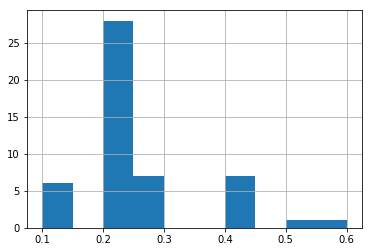In :
iris.iloc[:50, :].plot(x = 'Species', y=['SepalWidthCm', 'PetalWidthCm'], kind='hist')
plt.show()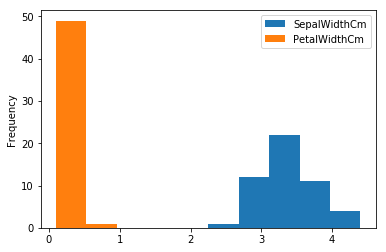Let's use the groupby function. This is another filtering application that allows users to group data by some category given in a column. For example, let's group the dataframe by species value and find their means:

In :
#Check values of the species
iris.Species.unique()

Out:
array(['Iris-setosa', 'Iris-versicolor', 'Iris-virginica'], dtype=object)
In :
iris.groupby('Species').mean()

Out:
Id SepalLengthCm SepalWidthCm PetalLengthCm PetalWidthCm
Species
Iris-setosa 25.5 5.006 3.418 1.464 0.244
Iris-versicolor 75.5 5.936 2.770 4.260 1.326
Iris-virginica 125.5 6.588 2.974 5.552 2.026
In :
##Can also find the median:
iris.groupby('Species').median().iloc[:, 1:]

Out:
SepalLengthCm SepalWidthCm PetalLengthCm PetalWidthCm
Species
Iris-setosa 5.0 3.4 1.50 0.2
Iris-versicolor 5.9 2.8 4.35 1.3
Iris-virginica 6.5 3.0 5.55 2.0
In :
#Use df.plot func again to plot the multiple y column values:
mean_groupby = (iris.groupby('Species').mean()).reset_index()
mean_groupby

Out:
Species Id SepalLengthCm SepalWidthCm PetalLengthCm PetalWidthCm
0 Iris-setosa 25.5 5.006 3.418 1.464 0.244
1 Iris-versicolor 75.5 5.936 2.770 4.260 1.326
2 Iris-virginica 125.5 6.588 2.974 5.552 2.026
In :
pd.DataFrame(mean_groupby.iloc[0, :]).T.plot.bar()
plt.show()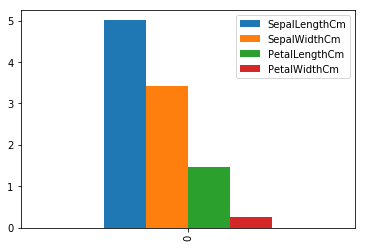In :
mean_groupby.plot(x = 'Species', y =['SepalLengthCm', 'SepalWidthCm'], kind='bar')
plt.show()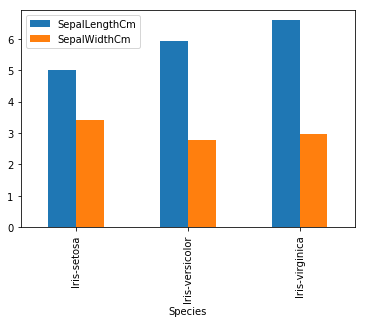In :
median_groupby = iris.groupby('Species').median()
median_groupby
#notice we can igonore the ID column

Out:
Id SepalLengthCm SepalWidthCm PetalLengthCm PetalWidthCm
Species
Iris-setosa 25.5 5.0 3.4 1.50 0.2
Iris-versicolor 75.5 5.9 2.8 4.35 1.3
Iris-virginica 125.5 6.5 3.0 5.55 2.0
In :
mean_groupby.plot(x = 'Species', y = ['PetalLengthCm', 'PetalWidthCm', 'SepalLengthCm', 'SepalWidthCm'], kind='bar')
plt.show()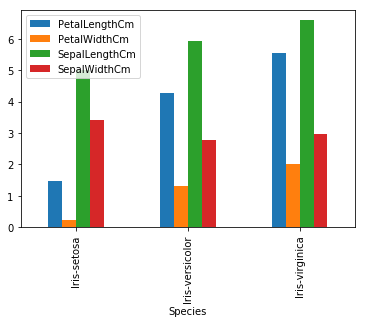In :
#Using the salaries dataset, let's make a bar chart showing salaries by position
#Again same concept for multiple columns in Y; however putting level doesn't make the graph clear since the Y axis limit is too large to show.
salaries.plot(x = 'Position', y=['Salary'], kind = 'Bar')
plt.show()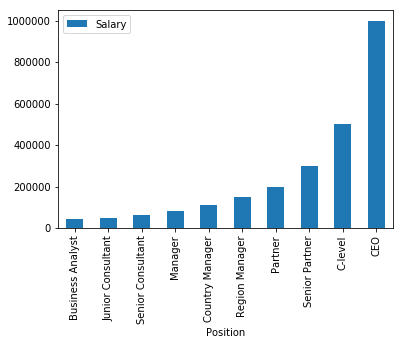## 2. Basic graphs with single/multiple plots & Using the Seaborn Package¶

### Anatomy of a figure¶

Figure: the outermost container of the graphic. One figure can hold multiple graphs. These graphs are known as Axes objects. A figure can be thought of as a basket of graphs(Axes objects). Axes: the individual plot (Not the same as axis !!). Title: Sort of obvious. Axis: X and Y axis X/Y label: labels for both x & y. Ticks are the increments. Spine: the line noting the data area boundaries

Let's first create a simple plot. Here we will tell python what to directly plot given 2 arrays that hold the X and Y values.

In :
plt.plot([1,2,3,4,5], [1,5,10,18, 22])
plt.show()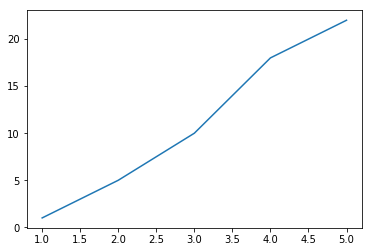Notice that Matplotlib automatically calibarates the points in both the X and Y axes to what it sees best. You may also customize the frequency of ticks, labels, and colors of the plot. A great article from Medium.com may explain this process of controlling the aesthetics of your figure: https://towardsdatascience.com/customizing-plots-with-python-matplotlib-bcf02691931f

In :
figure1, axes = plt.subplots(nrows=1,ncols=1, figsize = (12,5))
ax1 = plt.bar(np.arange(10), salaries.Salary)
plt.xticks(np.arange(10), salaries.Position, rotation = 25)
plt.show()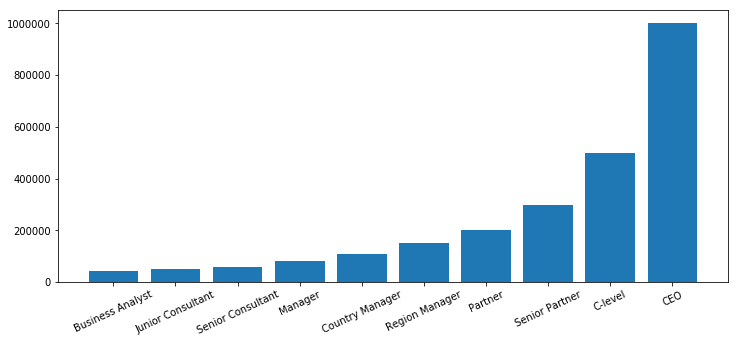Now we'll do the same with the Seaborn package!

In :
import seaborn as sns
sns.set_style("darkgrid")

figure1, axes = plt.subplots(nrows=1,ncols=1, figsize = (12,8))
axes = sns.barplot(x = salaries.Position, y = salaries.Salary, color = 'red')
axes.set_xticklabels(axes.get_xmajorticklabels(), fontsize=8, rotation = 10) #rotate the xticks in counterclockwise direction
axes.set_ylabel("Corresponding Salary")
figure1.suptitle("Salaries by Position", fontsize = 18) #The title of the figure
plt.show()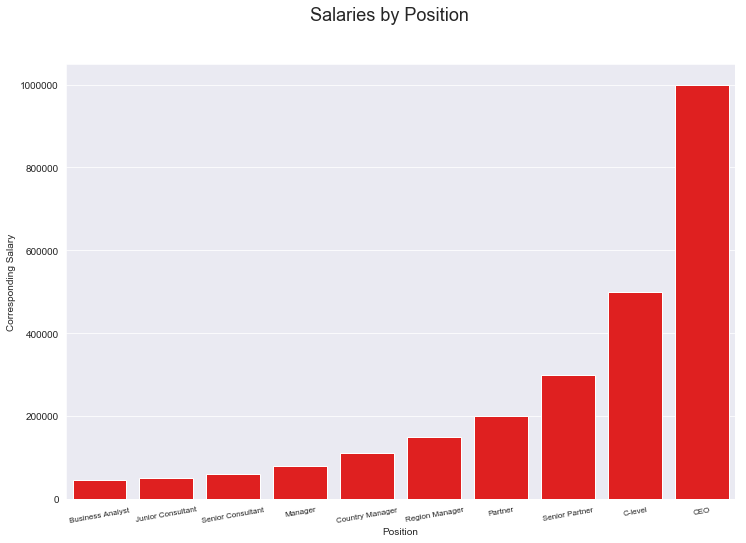Thus, this seems a bit more intuitive than the previous example. Note the changeable characteristics: size of xticks, x/y labels, figure title, color. Next, let's make a 2 subplots that each contain a scatterplot. We will be using the iris dataset for this problem.

In :
iris = pd.read_csv("Iris.csv")
figure2, axes = plt.subplots(nrows=1, ncols = 2, figsize = (8,5))
scatterplot1 = sns.scatterplot(iris.SepalLengthCm, iris.SepalWidthCm, ax= axes)
scatterplot2 = sns.scatterplot(iris.PetalLengthCm, iris.PetalWidthCm, ax = axes)
scatterplot1.set_title("Sepal") #Assigns an individial title for each subplot
scatterplot2.set_title("Petal")
figure2.suptitle("Comparing Widths/Lengths for Sepal/Petal")
plt.show()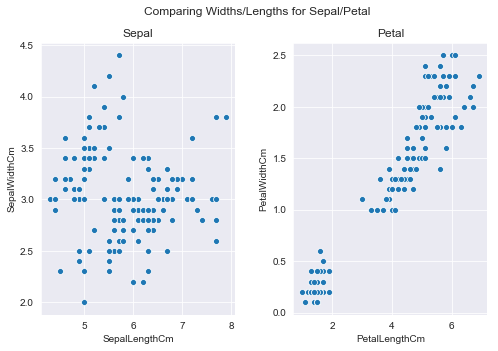Let's now make some boxplots. Our x-axis here will be categorical. The 'Species' column will serve as the categories. We can then compare the boxplot for each category with a given continious variable (such as sepal width/length or petal width/length). Let's make one graph that compares the petal length for each category.

In :
figure3, axes = plt.subplots(nrows = 1, ncols = 1, figsize = (8,5))
boxplot = sns.boxplot(x = "Species", y = "PetalLengthCm", data = iris, ax= axes)
boxplot.set_title("Boxplots for petal lengths by iris species")
plt.show()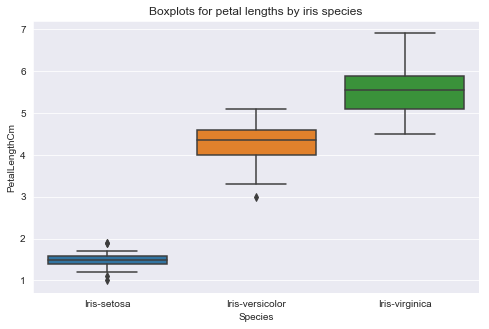Create four plots where each subplots compare boxplots by certain measure.

In :
figure4, axes = plt.subplots(nrows = 2, ncols = 2, figsize = (12,8))
boxplot1 = sns.boxplot(x = "Species", y = "PetalLengthCm", data = iris, ax= axes[0,0])
boxplot2 = sns.boxplot(x = "Species", y = "PetalWidthCm", data = iris, ax= axes[0,1])
boxplot3 = sns.boxplot(x = "Species", y = "SepalLengthCm", data = iris, ax= axes[1,0])
boxplot4 = sns.boxplot(x = "Species", y = "SepalWidthCm", data = iris, ax= axes[1,1])
plt.show()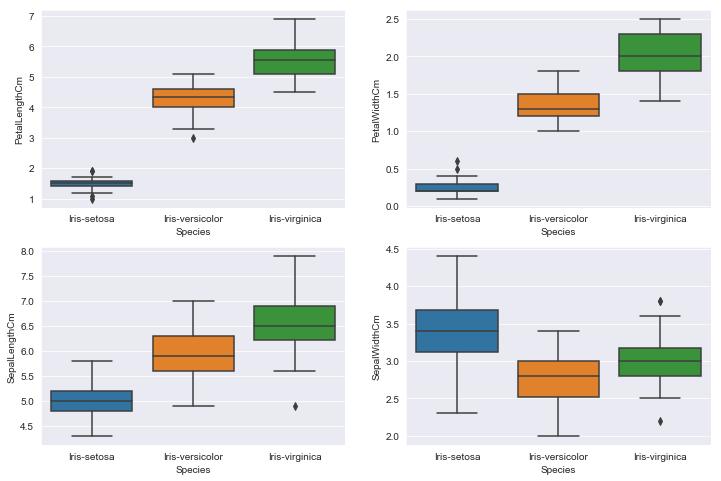Notice the pattern of creating these subplots and their particular syntax when choosing a certain plot. You may observe a reccuring pattern ("sns.{name of plot}"(Parameters...)). Keep in mind that this isn't the pattern for a lot of illustrations, these are just the basics. Let's make another similar figure but with violin plots.

In :
figure5, axes = plt.subplots(nrows = 2, ncols = 2, figsize = (12,8))
violinplot1 = sns.violinplot(x = "Species", y = "PetalLengthCm", data = iris, ax= axes[0,0])
violinplot2 = sns.violinplot(x = "Species", y = "PetalWidthCm", data = iris, ax= axes[0,1])
violinplot3 = sns.violinplot(x = "Species", y = "SepalLengthCm", data = iris, ax= axes[1,0])
violinplot4 = sns.violinplot(x = "Species", y = "SepalWidthCm", data = iris, ax= axes[1,1])
plt.show()

C:\Users\Brian\Anaconda3\lib\site-packages\scipy\stats\stats.py:1713: FutureWarning: Using a non-tuple sequence for multidimensional indexing is deprecated; use arr[tuple(seq)] instead of arr[seq]. In the future this will be interpreted as an array index, arr[np.array(seq)], which will result either in an error or a different result.
return np.add.reduce(sorted[indexer] * weights, axis=axis) / sumval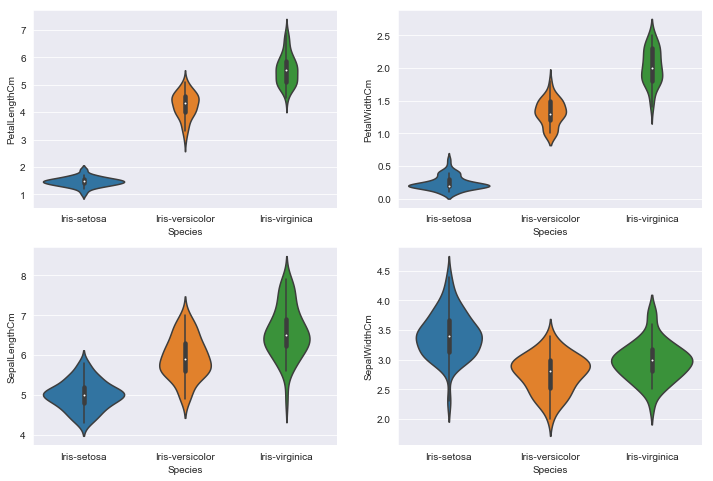### Other Seaborn Plots¶

In :
sns.distplot(iris['SepalLengthCm'], kde=True)
plt.show()

C:\Users\Brian\Anaconda3\lib\site-packages\scipy\stats\stats.py:1713: FutureWarning: Using a non-tuple sequence for multidimensional indexing is deprecated; use arr[tuple(seq)] instead of arr[seq]. In the future this will be interpreted as an array index, arr[np.array(seq)], which will result either in an error or a different result.
return np.add.reduce(sorted[indexer] * weights, axis=axis) / sumval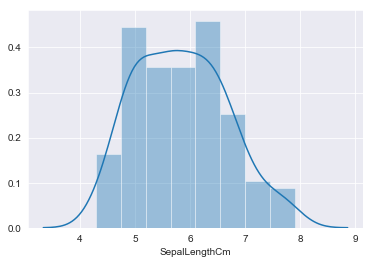Notice that for this figure we didn't type nor assigned a 'figure,ax' variable. Creating thesecan give you more customization - compare the creation of these variables and how we assigned for the scatterplots, boxplots, and violin plots.

The following graphs are also created without the two assignments.

In :
sns.barplot(x = 'Species', y='SepalLengthCm', data = mean_groupby)
plt.show()

#Similar to mean_groupby.plot(x = 'Species', y =['SepalLengthCm'], kind='bar')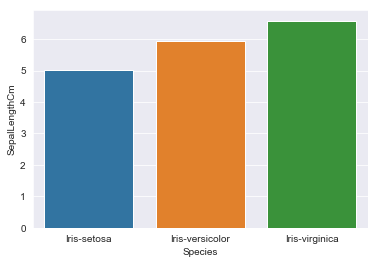In :
#Examples of some multivariate graphs:
sns.lmplot(x = 'PetalLengthCm', y = 'PetalWidthCm', data = iris, hue = 'Species', markers =['x', 'o', '*'])
plt.show()

C:\Users\Brian\Anaconda3\lib\site-packages\scipy\stats\stats.py:1713: FutureWarning: Using a non-tuple sequence for multidimensional indexing is deprecated; use arr[tuple(seq)] instead of arr[seq]. In the future this will be interpreted as an array index, arr[np.array(seq)], which will result either in an error or a different result.
return np.add.reduce(sorted[indexer] * weights, axis=axis) / sumval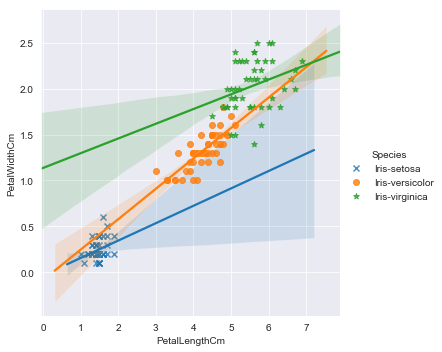In :
sns.lmplot(x = 'PetalLengthCm', y = 'PetalWidthCm', data = iris.iloc[:50, :], hue = 'Species')
plt.show()

C:\Users\Brian\Anaconda3\lib\site-packages\scipy\stats\stats.py:1713: FutureWarning: Using a non-tuple sequence for multidimensional indexing is deprecated; use arr[tuple(seq)] instead of arr[seq]. In the future this will be interpreted as an array index, arr[np.array(seq)], which will result either in an error or a different result.
return np.add.reduce(sorted[indexer] * weights, axis=axis) / sumval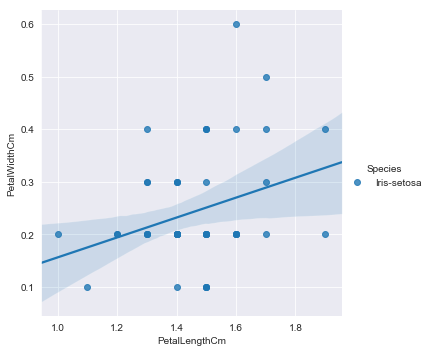In :
#Create a heatmap:
sns.heatmap(iris.iloc[:50, 1:5].corr(), annot=True)
plt.show()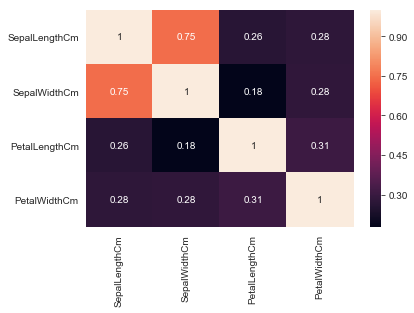There are tons of examples to follow from in the Seaborn tutorial webpage: https://seaborn.pydata.org/examples/index.html Furthermore, understanding the basics of matplotlib are so important! A more extensive tutorial in understanding the figure and plotting the area of the figure can be found here: https://matplotlib.org/tutorials/introductory/pyplot.html.